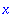Error: cannot assume on a constant object

This error occurs when the first argument to assume or additionally contains no names upon which to make assumptions. The most common cause is an attempt to make an assumption about a variable that has already been assigned a constant value. It doesn't matter whether or not this value has the property being assumed.

> x:= 3:
assume(x > 0);

Error, (in assume) cannot assume on a constant object

Like most procedures, assume begins by evaluating its arguments. This replaces the variable x by the value assigned to that variable, so the effect is the same as if you typed assume(3 > 0) . It happens to be true, but that's irrelevant: it doesn't say anything about any variables, and therefore it results in an error message.

It is possible to use quotes to avoid having the variable replaced by its value:

> assume('x' > 0);

However, this removes the previously assigned value from the variable.

> x;